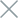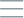• Home
• Tags
• Series
•# Greedy Search Algorithm in PHP: Explanation, Example & Code

The Greedy Search Algorithm is a significant approach in PHP programming, used to address optimization problems by making decisions based on short-term benefits. This algorithm is commonly applied in optimization challenges, job scheduling, and optimal configurations.

## How the Greedy Search Algorithm Works

The Greedy Search Algorithm focuses on making decisions based on short-term benefits without considering long-term impacts. It involves the following steps:

1. Identify Optimization Task: The algorithm identifies the task to be optimized and available options for selection.
2. Make Decision: The algorithm makes decisions based on short-term benefits, such as selecting an option that provides the highest immediate value.
3. Check Termination Condition: The algorithm checks whether the termination condition is met or the final selection is made. If not, the process continues.

• Effective for Large Problems: This algorithm is often efficient when dealing with problems that require quick decisions and don't need to consider all options.
• Easy to Implement: The Greedy Search Algorithm is generally easy to implement and doesn't require significant computational resources.

• Lack of Global Optimization Guarantee: This algorithm may lead to locally optimal solutions that are not globally optimal.
• Disregard for Long-Term Impact: The algorithm overlooks the long-term impacts of decisions and focuses only on short-term benefits.

## Example and Explanation

Consider an example of a simple job scheduling problem: Finding the optimal schedule to complete the maximum number of jobs within a fixed time frame using the Greedy Search Algorithm in PHP.

``````function greedyScheduler(\$jobs, \$timeLimit) {
// Implementation of greedy scheduling algorithm
// ...
}

\$jobs = array(
array('Job A', 4),
array('Job B', 2),
array('Job C', 5),
array('Job D', 3)
);

\$timeLimit = 10;

\$schedule = greedyScheduler(\$jobs, \$timeLimit);
echo "Optimal schedule: ";
foreach (\$schedule as \$job) {
echo \$job . " ";
}
``````

In this example, we use the Greedy Search Algorithm to schedule jobs in a way that maximizes the number of jobs completed within a fixed time frame. The algorithm selects jobs based on the shortest execution time. The result is a schedule where each job is added one by one in the order of shortest execution time.

While this example demonstrates how the Greedy Search Algorithm can be used to solve a job scheduling problem, it can also be applied to other optimization problems in PHP, such as resource optimization or configuration management.